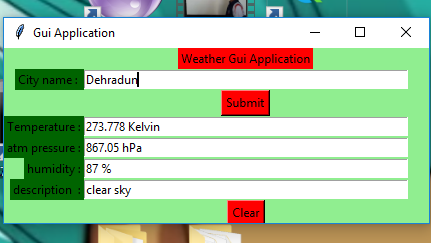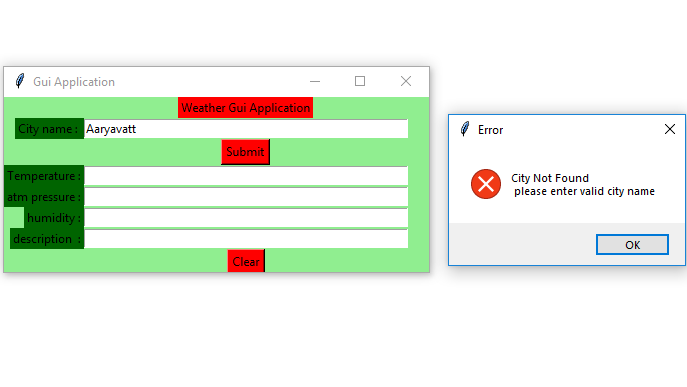# Python | Real time weather detection using Tkinter

• Difficulty Level : Hard
• Last Updated : 10 Jun, 2021

Prerequisites : Introduction to tkinter | Find current weather of any city
Python offers multiple options for developing GUI (Graphical User Interface). Out of all the GUI methods, tkinter is the most commonly used method. It is a standard Python interface to the Tk GUI toolkit shipped with Python. Python with tkinter outputs the fastest and easiest way to create the GUI applications. Now, it’s upto the imagination or necessity of developer, what he/she want to develop using this toolkit.
To create a tkinter :

• Importing the module – tkinter
• Create the main window (container)
• Add any number of widgets to the main window.
• Apply the event Trigger on the widgets.

Let’s create a GUI based simple Real-time Weather detection application which can give Weather details of any cities.
Modules required:

```tkinter
requests
json```

Below is the implementation :

## Python3

 `# import all functions from the tkinter ``from` `tkinter ``import` `*` `from` `tkinter ``import` `messagebox` `# function to find weather details``# of any city using openweathermap api``def` `tell_weather() :` `    ``# import required modules``    ``import` `requests, json` `    ``# enter your api key here``    ``api_key ``=` `"Your_API_key"` `    ``# base_url variable to store url``    ``base_url ``=` `"http://api.openweathermap.org/data/2.5/weather?"`  `    ``# take a city name from city_field entry box``    ``city_name ``=` `city_field.get()` `    ``# complete_url variable to store complete url address``    ``complete_url ``=` `base_url ``+` `"appid ="` `+` `api_key``                             ``+` `"&q ="` `+` `city_name` `    ``# get method of requests module``    ``# return response object``    ``response ``=` `requests.get(complete_url)` `    ``# json method of response object convert``    ``# json format data into python format data``    ``x ``=` `response.json()` `    ``# now x contains list of  nested dictionaries``    ``# we know dictionary contains key value pair``    ``# check the value of "cod" key is equal to "404"``    ``# or not if not that means city is found``    ``# otherwise city is not found``    ``if` `x[``"cod"``] !``=` `"404"` `:` `        ``# store the value of "main" key in variable y``        ``y ``=` `x[``"main"``]` `        ``# store the value corresponding to the "temp" key of y``        ``current_temperature ``=` `y[``"temp"``]` `        ``# store the value corresponding to the "pressure" key of y``        ``current_pressure ``=` `y[``"pressure"``]` `        ``# store the value corresponding to the "humidity" key of y``        ``current_humidity ``=` `y[``"humidity"``]` `        ``# store the value of "weather" key in variable z``        ``z ``=` `x[``"weather"``]` `        ``# store the value corresponding to the "description" key``        ``# at the 0th index of z``        ``weather_description ``=` `z[``0``][``"description"``]` `        ``# insert method inserting the``        ``# value in the text entry box.``        ``temp_field.insert(``15``, ``str``(current_temperature) ``+` `" Kelvin"``)``        ``atm_field.insert(``10``, ``str``(current_pressure) ``+` `" hPa"``)``        ``humid_field.insert(``15``, ``str``(current_humidiy) ``+` `" %"``)``        ``desc_field.insert(``10``, ``str``(weather_description) )` `    ``# if city is not found                  ``    ``else` `:` `        ``# message dialog box appear which``        ``# shows given Error message``        ``messagebox.showerror(``"Error"``, ``"City Not Found \n"``                             ``"Please enter valid city name"``)` `        ``# clear the content of city_field entry box``        ``city_field.delete(``0``, END)`  `# Function for clearing the``# contents of all text entry boxes ``def` `clear_all() :``    ``city_field.delete(``0``, END) ``    ``temp_field.delete(``0``, END)``    ``atm_field.delete(``0``, END)``    ``humid_field.delete(``0``, END)``    ``desc_field.delete(``0``, END)` `    ``# set focus on the city_field entry box``    ``city_field.focus_set()`  `# Driver code``if` `__name__ ``=``=` `"__main__"` `:` `    ``# Create a GUI window``    ``root ``=` `Tk()` `    ``# set the name of tkinter GUI window``    ``root.title(``"Gui Application"``)` `    ``# Set the background colour of GUI window``    ``root.configure(background ``=` `"light green"``)` `    ``# Set the configuration of GUI window``    ``root.geometry(``"425x175"``)` `    ``# Create a Weather Gui Application label``    ``headlabel ``=` `Label(root, text ``=` `"Weather Gui Application"``,``                      ``fg ``=` `'black'``, bg ``=` `'red'``)``    ` `    ``# Create a City name : label``    ``label1 ``=` `Label(root, text ``=` `"City name : "``,``                   ``fg ``=` `'black'``, bg ``=` `'dark green'``)``    ` `    ``# Create a City name : label``    ``label2 ``=` `Label(root, text ``=` `"Temperature :"``,``                   ``fg ``=` `'black'``, bg ``=` `'dark green'``)` `    ``# Create a atm pressure : label``    ``label3 ``=` `Label(root, text ``=` `"atm pressure :"``,``                   ``fg ``=` `'black'``, bg ``=` `'dark green'``)` `    ``# Create a humidity : label``    ``label4 ``=` `Label(root, text ``=` `"humidity :"``,``                   ``fg ``=` `'black'``, bg ``=` `'dark green'``)` `    ``# Create a description :label``    ``label5 ``=` `Label(root, text ``=` `"description  :"``,``                   ``fg ``=` `'black'``, bg ``=` `'dark green'``)``    `  `    ``# grid method is used for placing``    ``# the widgets at respective positions``    ``# in table like structure . ``    ``headlabel.grid(row ``=` `0``, column ``=` `1``)``    ``label1.grid(row ``=` `1``, column ``=` `0``, sticky ``=``"E"``)``    ``label2.grid(row ``=` `3``, column ``=` `0``, sticky ``=``"E"``)``    ``label3.grid(row ``=` `4``, column ``=` `0``, sticky ``=``"E"``)``    ``label4.grid(row ``=` `5``, column ``=` `0``, sticky ``=``"E"``)``    ``label5.grid(row ``=` `6``, column ``=` `0``, sticky ``=``"E"``)`  `    ``# Create a text entry box``    ``# for filling or typing the information.``    ``city_field ``=` `Entry(root)``    ``temp_field ``=` `Entry(root)``    ``atm_field ``=` `Entry(root)``    ``humid_field ``=` `Entry(root)``    ``desc_field ``=` `Entry(root)` `    ``# grid method is used for placing``    ``# the widgets at respective positions``    ``# in table like structure .``    ``# ipadx keyword argument set width of entry space .``    ``city_field.grid(row ``=` `1``, column ``=` `1``, ipadx ``=``"100"``)``    ``temp_field.grid(row ``=` `3``, column ``=` `1``, ipadx ``=``"100"``)``    ``atm_field.grid(row ``=` `4``, column ``=` `1``, ipadx ``=``"100"``)``    ``humid_field.grid(row ``=` `5``, column ``=` `1``, ipadx ``=``"100"``)``    ``desc_field.grid(row ``=` `6``, column ``=` `1``, ipadx ``=``"100"``)` `    ``# Create a Submit Button and attached``    ``# to tell_weather function``    ``button1 ``=` `Button(root, text ``=` `"Submit"``, bg ``=` `"red"``,``                     ``fg ``=` `"black"``, command ``=` `tell_weather)` `    ``# Create a Clear Button and attached``    ``# to clear_all function``    ``button2 ``=` `Button(root, text ``=` `"Clear"``, bg ``=` `"red"``,``                     ``fg ``=` `"black"``, command ``=` `clear_all)` `    ``# grid method is used for placing``    ``# the widgets at respective positions``    ``# in table like structure .``    ``button1.grid(row ``=` `2``, column ``=` `1``)``    ``button2.grid(row ``=` `7``, column ``=` `1``)``    ` `    ``# Start the GUI``    ``root.mainloop()`

Output :My Personal Notes arrow_drop_up